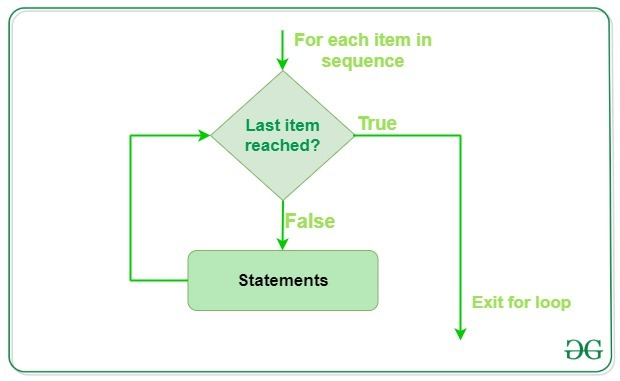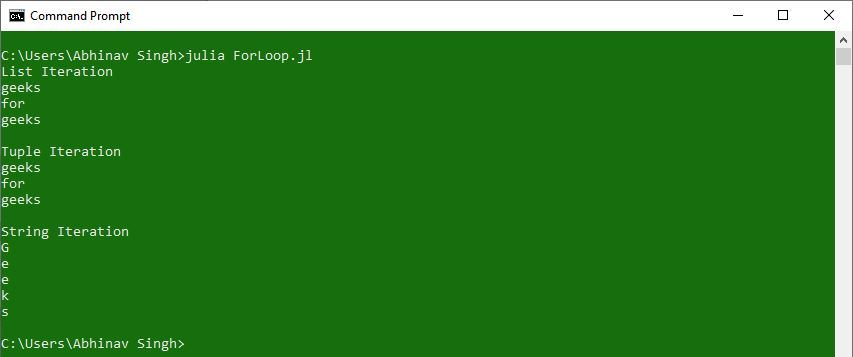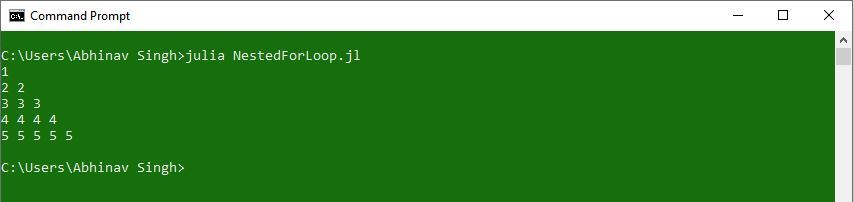# For loop in Julia

• Last Updated : 19 Feb, 2020

For loops are used to iterate over a set of values and perform a set of operations that are given in the body of the loop. For loops are used for sequential traversal. In Julia, there is no C style for loop, i.e., for (i = 0; i < n; i++). There is a "for in" loop which is similar to for each loop in other languages. Let us learn how to use for in loop for sequential traversals.Syntax:

```for iterator in range
statements(s)
end
```

Here, ‘for‘ is the keyword stating for loop, ‘in‘ keyword is used to define a range in which to iterate, ‘end‘ keyword is used to denote the end of for loop.

Example:

 `# Julia program to illustrate ``# the use of For loop`` ` `print``(``"List Iteration\n"``) ``l ``=` `[``"geeks"``, ``"for"``, ``"geeks"``] ``for` `i ``in` `l``    ``println(i) ``end`` ` `# Iterating over a tuple (immutable) ``print``(``"\nTuple Iteration\n"``) ``t ``=` `(``"geeks"``, ``"for"``, ``"geeks"``) ``for` `i ``in` `t``    ``println(i) ``end`` ` `# Iterating over a String ``print``(``"\nString Iteration\n"``)     ``s ``=` `"Geeks"``for` `i ``in` `s ``    ``println(i) ``end`

Output:Nested for-loop: Julia programming language allows to use one loop inside another loop. The following section shows an example to illustrate the concept.
Syntax:

```for iterator in range
for iterator in range
statements(s)
statements(s)
end
end
```

Example:

 `# Julia program to illustrate ``# the use of Nested For-Loops`` ` `# Outer For-loop``for` `i ``in` `1``:``5` ` ` `    ``# Inner For-loop``    ``for` `j ``in` `1``:i ``         ` `        ``# Print statement``        ``print``(i, ``" "``)``    ``end``    ``println() ``end`

Output:My Personal Notes arrow_drop_up